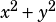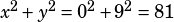Hi Mike, sorry for asking questions so frequently. I’m on my 16th practice test now and I am amazed to find something new everytime. Anyway…

If x and y are numbers such that (x+9)(y-9)=0 what is the smallest possible value of X^2 + y^2

A 0
B 9
C 18
D 81
E 162

I got E when I did this but the answer is 81.Why?

If (x + 9)(y – 9) = 0, then either x = –9 or y = 9, but those don’t BOTH have to be true. To minimize, assume that one of them is equal to 0.

For example, say x = 0, and y = 9. Then (x + 9)(y – 9) = 0, and.